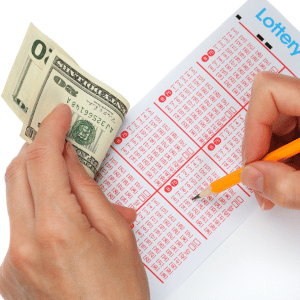# EuroMillions: How Many Number Combinations Are Possible?

EuroMillions is one of the most popular and widely played lotteries across Europe, captivating the hopes and dreams of millions. Players often wonder about the vast array of possible number combinations within this multi-national lottery. The question that frequently arises is: “How many number combinations are there in EuroMillions?”

## Understanding EuroMillions Number Combinations

The EuroMillions lottery involves selecting five main numbers from a pool of 1 to 50 and two Lucky Star numbers from a pool of 1 to 12. To determine the total number of combinations, we can use mathematical calculations.

To find the number of combinations of the main numbers, we use the formula for combinations: nCr = n! / (r! * (n – r)!), where “n” is the total numbers available and “r” is the quantity of numbers being chosen.

For the main numbers in EuroMillions, we have 50 numbers to choose from and we need to choose 5. Therefore, the calculation for the combinations of the main numbers is 50C5 = 2,118,760.

Similarly, for the Lucky Star numbers, there are 12 numbers and we need to choose 2. Using the combination formula, the number of combinations for the Lucky Star numbers is 12C2 = 66.

## Total CombinationsTo determine the total number of combinations in EuroMillions, we multiply the combinations of the main numbers by the combinations of the Lucky Star numbers. Therefore, 2,118,760 (main numbers) * 66 (Lucky Star numbers) = 139,838,160.

Hence, in the EuroMillions lottery, there are a staggering 139,838,160 possible number combinations that could be drawn. This vast number of potential combinations underlines the remarkable odds of winning the jackpot.

## Odds of Winning

Considering the extensive number of combinations available, the odds of any single ticket matching the five main numbers along with the two Lucky Star numbers are notably slim. However, this doesn’t deter the enthusiasm of players who engage in the lottery in pursuit of life-altering winnings.

The chances of hitting the jackpot stand at approximately 1 in 139.8 million, based on these astronomical number combinations. To put this into perspective, it’s crucial to understand that the odds are stacked against an individual player but it’s the thrill of the possibility that keeps the excitement alive for many participants.

## Strategies and Perceptions

Some players opt for various strategies in selecting numbers, including birthdays, anniversaries, or random selections, while others utilize statistical analysis of previous draws to make their choices. However, it’s important to recognize that in a game of chance such as EuroMillions, each combination has an equal chance of being drawn, irrespective of its perceived uniqueness.

While the mathematical calculations unveil the enormous quantity of possible combinations, it’s crucial to acknowledge that every combination has the same probability of being drawn, making the lottery a pure game of chance.

In conclusion, the EuroMillions lottery boasts an incredible number of combinations, creating significant odds for players aiming to hit the jackpot. Understanding the sheer scale of number combinations sheds light on the game’s nature, where luck plays an undeniable role in altering lives forever.